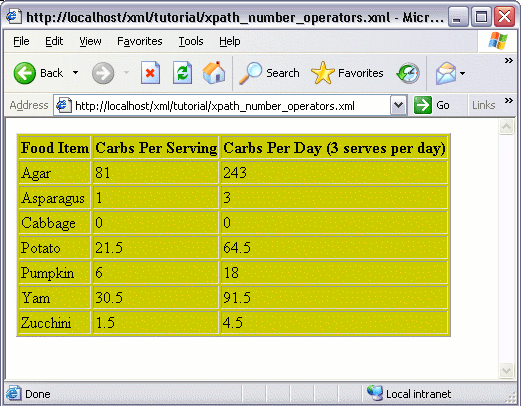# XPath Number Operators

XPath includes a range of operators you can take advantage of when using number values within your expressions.

## Number Operators

Here's a list of number operators you can use in your XPath expressions:

OperatorDescription
-Used for subtraction.
*Used for multiplication.
divUsed for division.
modReturns the modulus of two numbers. (The modulus is the remainder after you divide the two numbers).

## Number Functions

Here's a list of functions you can use with numbers in your XPath expressions:

FunctionDescription
ceiling()Returns the smallest integer that is larger than the value provided.
floor()Returns the largest integer that is smaller than the value provided.
round()Rounds the value provided to the nearest integer.
sum()Returns the sum of the two numbers provided.

## Usage Example

### The Source XML File

We'll use the following XML file, which contains a list of vegetables and their associated nutritional value:

### The Requirement

This time, for each record, we'll display the carbohydrate value ("carbs"). We will also display a column that multiplies the number of carbs by 3 (representing 3 meals per day). Like this:### The Solution

We could modify the code from the previous lesson so that only the top 5 records are selected. We could achieve this using the XPath `<=` operator (along with the `position()` function), as follows: